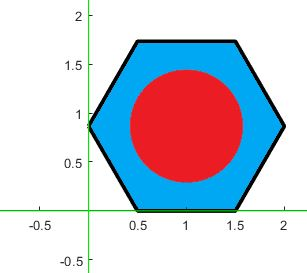# Problem 49943. Splitting Hexagon - Problem the third

Consider a hexagon sitting in Quadrant I as depicted in an example below:This hexagon is to be split into two regions (e.g., red and blue). Given the ratio between the two regions and the side of the hexagon, determine the radius of the circle that splits the region. The ratio between the regions (red to blue) is presented through the first two entries in the input. For example, if the ratio is 1 to 2, then these two numbers will be the first two entries in the input. The last entry is the side of the hexagon.

### Solution Stats

64.81% Correct | 35.19% Incorrect
Last Solution submitted on Mar 07, 2023

### Community Treasure Hunt

Find the treasures in MATLAB Central and discover how the community can help you!

Start Hunting!